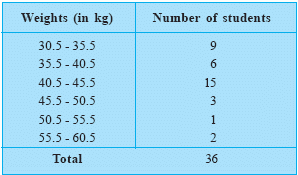Email us to get an instant 20% discount on highly effective K-12 Math & English kwizNET Programs!

#### Online Quiz (WorksheetABCD)

Questions Per Quiz = 2 4 6 8 10

### Middle/High School Algebra, Geometry, and Statistics (AGS)9.14 Graphical Representation of Statistical Data - Histograms

#### Histogram

It is graphical representation of frequency distribution in the form of rectangles with class intervals as bases and the corresponding frequencies as heights, there being no gap between any two successive rectangles.

In drawing a histogram of a given frequency distribution, we take the following steps:

Step 1: If the frequency table is in the inclusive form, we first convert it into an exclusive form and make it a continuous interval.
Step 2: Taking a suitable scale, we represent class limits along x axis.
Step 3: Taking a suitable scale, we represent frequency along y axis.
Step 4: We construct rectangles with bases along x axis and heights along y axis.
Step 5: Area of the rectangles so constructed should be proportional to the frequencies.

 Data in the tabular form is as follows Histogram representing the dataDirections: On the basis of the given information answer the following questions
 Q 1: The data is divided into how many class intervals?5476 Q 2: What is the area of the rectangle of the first class interval?50904530 Q 3: The class interval with highest frequency has how many entries more than the class interval with the lowest frequency12141315 Q 4: Which class interval has the lowest frequency?45.5 - 50.540.5 - 45.555.5 - 60.550.5 - 55.5 Question 5: This question is available to subscribers only! Question 6: This question is available to subscribers only!